Rudy Rucker's Kappatau Paper

Home

Biography

Books

Classes

Email

## How Flies Fly: Kappatau Space Curves

by Rudy Rucker
Department of Mathematics and Computer Science,
San Jose State University, San Jose CA 95192
www.rudyrucker.com

Copyright (C) Rudy Rucker 1999

Appeared in David Wolfe & Tom Rodgers, eds., Puzzlers' Tribute: A Feast for the Mind, A.K.Peters, Natick, MA, 2002

It's interesting to watch flies buzz around. They trace out curves in space that are marvelously three-dimensional. Birds fly along space curves too, but their airy swoops are not nearly so bent and twisted as are the paths of flies.

Is there a mathematical language for talking about the shapes of curves in space? Sure there is. Math is the science of form, and mathematicians are always studying nature for new forms to talk about.

Historically, space curves were first discussed by the mathematician Alexis-Claude Clairaut in a paper called "Recherche sur les Courbes a Double Courbure," published in 1731 when Clairaut was eighteen . Clairaut is said to have been an attractive, engaging man; he was a popular figure in eighteenth-century Paris society.

In speaking of "double curvature," Clairaut meant that a path through three-dimensional space can warp itself in two independent ways; he thought of a curve in terms of its shadow projections onto, say, the floor and a wall. In discussing the bending of the planar, "shadow" curves, Clairaut drew on recent work by the incomparable Isaac Newton.

Newton's mathematical curvature measures a curve's tendency to bend away from being a straight line. The more the curve bends, the greater is the absolute value of its curvature. From the viewpoint of a point moving along the curve, the curvature is said to be positive when the curve bends to the left, and negative when the curve bends to the right. The size of the curvature is determined by the principle that a circle of radius R should have curvature of 1/R. The smaller the radius, the greater the curvature. Figure 1 shows some examples of circular arcs.#### Figure 1: Curvature along circular arcs in the plane.

We often represent a curve in the plane by an equation involving x and y coordinates. Most calculus students remember a brief, nasty encounter with Newton's formula for the curvature of a curve; the formula uses fractional powers and the first and second derivatives of y with respect to x. Fortunately, there is no necessity for us to trundle out this cruel, ancient idol. Instead we think of curvature as a primitive notion and express the curve in a more natural way.

The idea is that instead of talking about positions relative to an arbitrary x axis and y axis, we think of a curve as being a bent number-line by itself. The curve is marked off in units of "arclength", where arclength is the distance measured along the curve, just as if the curve were a piece of rope that you could stretch out next to a ruler.

In this context, the most natural way to describe a plane curve is by an equation that gives the curvature directly as a function of arclength, an equation of the form kappa = f(s), where s stands for arclength and kappa is the commonly used symbol for curvature. Figure 2 shows two famous plane curves which happen to have simple expressions for curvature as a function of arclength. The catenary curve is the shape assumed by a chain (or bridge cable) suspended from two points, while the logarithmic spiral is a form very popular among our friends the molluscs.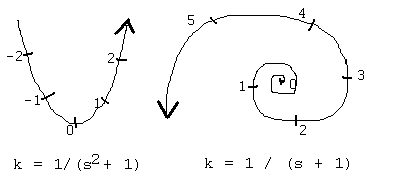#### Figure 2: The catenary and the logarithmic spiral expressed by natural equations, with curvature kappa a function of arclength s.

Note that for the spiral, the center is where s approaches -1; if you jump over the anomalous central point and push down into larger negative values of s, you produce a mirror-image of the spiral.

It would be nice to also think of space curves in a natural, coordinate-free way --- surely this is the way a fly buzzing around in the center of an empty room must think. Profound mathematical insights come hard, and it was a hundred and twenty years after Clairaut before the correct way to represent a space curve by intrinsic natural equations was finally discovered --- by the French mathematicians Joseph Alfred Serret and Frederic-Jean Frenet.

The idea is that at each point of a space curve one can define two numerical quantities called curvature and torsion. The curvature of a space curve is essentially the same as the curvature of a plane curve: it measures how rapidly the curve is bending to one side. The torsion measures a curve's tendency to twist out of a plane. But what exactly is meant by "bend to one side," and "twist out of a plane"? Which plane?

The idea is that at each point P of a space curve you can define three mutually perpendicular unit-length vectors: the tangent T, the normal N, and the binormal B. T shows the direction the curve is moving in, N lies along the direction which the curve is currently bending in, and B is a vector perpendicular to T and N. (In terms of the vector cross product, T cross N is B, N cross B is T, and B cross T is N.) For space curves we ordinarily work only with positive values of curvature, and have N point in the direction in which the curve is actually bending. (In certain of the analytical curves we’ll look at later we relax this condition and allow negative curvature of space curves.)

Taken together, T, N and B make up the so-called "moving trihedron of a space curve". In Figure 3 we show part of a space curve (actually a helix) with several instances of the moving trihedron. So that it's easier to see the three-dimensionality of the image, we draw the curve as a ribbon like a twisted ladder. The curve runs along one edge of the ladder, and the rungs of the ladder correspond to the directions of successive normals to the curve.#### Figure 3: The moving trihedron of a space curve: T the tangent, N the normal, and B the binormal.

To understand exactly how the normal is defined, it helps to think of the notion of the "osculating" (kissing) plane. At each point of a space curve there is some plane that best fits the curve at that point. The tangent vector T lies in this plane, and the direction perpendicular to T in this plane holds the normal N. The binormal is a vector perpendicular to the osculating plane.

With the idea of the moving trihedron in mind, we can now say that the curvature measures the rate at which the tangent turns, and the torsion measures the rate at which the binormal turns.

Note that T, N and B are always selected so as to form a right-handed coordinate system. This means that if you hold out the thumb, index finger and middle finger of your right hand, these directions correspond to the tangent, the normal, and the binormal.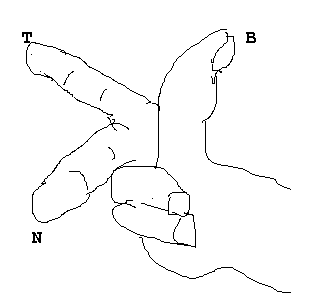#### Figure 4: A right-hand as a trihedron.

Just as the circle is the plane curve characterized by having constant curvature, the helix is the space curve characterized by having constant curvature and constant torsion. Figure 5 shows how the signs of the curvature and torsion affect the shapes of plane and space curves.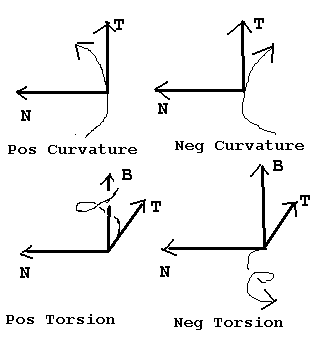#### Figure 5: How the signs of the curvature and torsion affect the motion of a curve.

Now let's look for some space formulae analogous to the plane formula stating that the curvature of a circle of radius R is 1/R. Think of a helix as wrapping around a cylinder --- like a vine growing up a post. Let R be the radius of the cylinder, and let H represent the turn-height: the vertical distance it takes the helix to make one complete turn (and to make the formulae nicer, we measure turn-height in units 2*pi as large as the units we measure R in.)

The sizes of the curvature and torsion on a helix with radius R and turn-height H are given by two nice equations. We write "tau" for torsion and, as before, "kappa" for curvature:

kappa = R / (R^2 + H^2), and

tau = H / (R^2 + H^2).

It's an interesting exercise in algebra to try and turn these two equations around and solve for R and H in terms of kappa and tau. (Hint: Start by computing kappa^2 + tau^2. When you're done, your new equations will look a lot like the original equations.)

Some initial things to notice are that if H is much smaller than R, you get a curvature roughly equal to 1/R, just like for a circle, and a tau very close to 0. If, on the other hand, R is very close to zero, then the torsion is roughly 1/H while the curvature is close to 0. A fly which does a barrel-roll while moving through a nearly straight distance of H has a torsion of 1/H. The faster it can roll, the greater is its torsion.

A less obvious fact is that if we look down on a plane showing all possible positive combinations R and H, the lines of constant curvature lie on horizontal semi-circles; while the points representing constant torsion lie on vertical semi-circles. The curvature and torsion combinations gotten by stretching a given Slinky lie along a quarter circle centered on the origin. Apparently the two families of semi-circles are perpendicular to each other.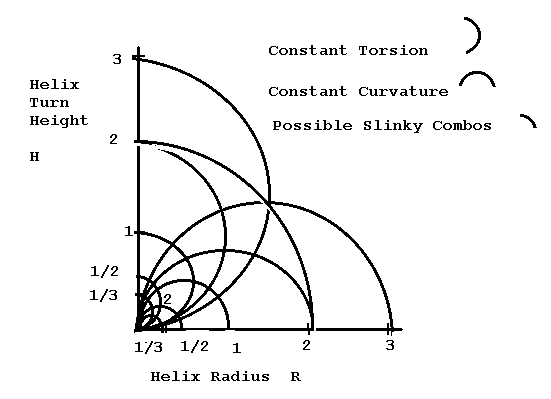#### Figure 6: Lines of constant curvature and torsion for combinations of R and H.

Suppose I have a helix like a steel Slinky spring. What happens to the curvature and the torsion as I stretch a single turn of it without untwisting? Suppose that the initial radius of the helix is A. Given the physical fact that the length of one twist of the Slinky keeps the same length, you can show that as you stretch it, R^2 + H^2 will stay constant at a value of A^2, which corresponds to a circle of radius A around the origin of the R-H plane. As you stretch a Slinky loop with the particular starting radius of 2, its R and H values will move along the dotted blue line shown in Figure 6. Figure 7 shows what a few of the intermediate positions will look like. Curvature is being traded off for torsion.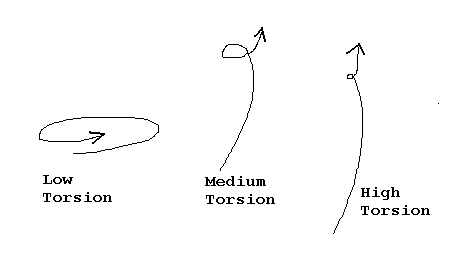#### Figure 7: Stretching a Slinky turns curvature into torsion.

Here's another algebra problem: If you know that R^2 + H^2 = A^2, what can you say about the sum kappa^2 + tau^2?

One fact that seems odd at first is that the curvature and torsion of a helix are dependent on the size of the helix. If you make both R and H five times as big, you make the torsion and curvature 1/10 as big. If you make R and H N times as big, you make the curvature and torsion 1/(2*N) as big.

But this makes sense if you think of a fly that switches from a small helix to a big helix; it is indeed changing the way that its flying, so it makes sense that the kappa and the tau should change.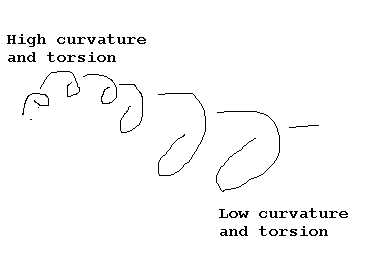#### Figure 8: Changing Curvature and Torsion.

This observation suggests a simple way to express the difference between flies and birds --- flies fly with much higher curvature and torsion than do the birds. Gnats, for that matter, fly even more tightly knotted paths, and have very large values of curvature and torsion.

Just as in the plane, a space curve can be specified in terms of natural equations that give the curvature and torsion as functions of the arclength. These equations have the form kappa = f(s) and tau = g(s). The shape and size of the space curve is uniquely determined by the curvature and the torsion functions. Figures 9 and 10 shows two intriguing space curves given by simple curvature and torsion functions.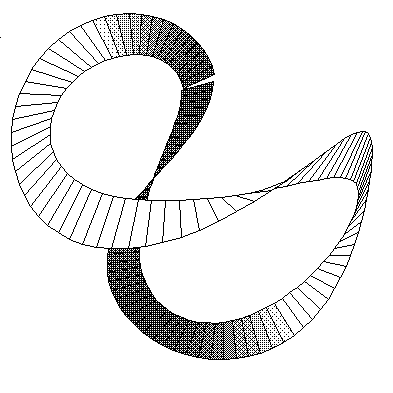#### Figure 9: The rocker, with natural equations kappa = 1 and tau = sine(arclength)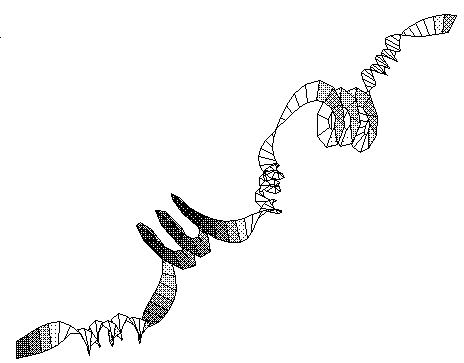#### Figure 10: The phone-cord, with natural equations kappa = sine(arclength) and tau = 1.

Well, actually I used kappa = 10*sine(arclength) and tau = 3 to make the picture look better. Note that this is a space curve where we do allow ourselves to put in negative values for the curvature.

There is not a large literature on these "kappatau" curves, so I've given my own names to these two: the rocker, and the phone-cord.

At one time I thought that the rocker was a correct way to represent the seam on a tennis-ball or the stitching on a baseball, but helpful email from the great mathematician John Horton Conway convinced me I was wrong. Conway makes the anthropological conjecture that every time a mathematician discovers a curve that he or she thinks might be the true baseball curve, the curve is a different one.

An analysis of the real-world baseball stitch curve can be found in the web-published paper "Designing A Baseball Cover by Richard Thompson of the Department of Mathematics, University of Arizona . It turns out the baseball stitch curve is based on something so prosaic as a patented 1860s pen and ink drawing of a plane shape used to cut out the leather for a half of a baseball, a shape arrived at by trial and error. Thompson finds a fairly gnarly closed-form approximation of this shape.

Not only does my rocker fail to match the baseball stitch curve, it can be proved that the rocker curve does not in fact lie on the surface of a sphere (even though it kind of looks like it does). It fails to satisfy the following necessary condition for lying on the surface of the sphere, where s stands for arclength (see ).

d/ds[(1/tau)*d/ds(1/kappa)] + tau*(1/kappa) = 0

(For kappa = 1 and tau = sin(s), the left-hand side of this is sin(s), which isn’t 0.)

Numerical estimates indicate that the arclength of the rocker has exactly twice the length of a circle of the same radius. This suggests an easy way to make a rocker. Cut out two identical annuli (thick circles) from some fairly stiff paper (manila file folders are good), cut radial slits in the annuli, tape two of the slit-edges together, bend the annuli in two different ways (one like a clockwise helix and one like a counterclockwise helix) and tape the other two slit-edges together, forming a continuous band of double length . Because an annulus cannot bend along its osculating plane, the curvature of the shape is fixed along the arclength. Because half the band is like a clockwise helix and half is like a counterclockwise helix, when the shape relaxes, the torsion presumably varies with the arclength like a sine wave function that goes between plus one and minus one. The torsion seems to be zero at the two places where the slits are taped together. Note that I have not proved that my empirical paper rocker is the same as my mathematical rocker, this is simply my conjecture.#### Figure 11: Make your own rocker.

• To make the rocker, make a (larger) copy of Figure 11 on stiff paper.
• Cut along all solid lines.
• Tape edge A to edge B* with the letters on the same side.
• Bend the two rings in the opposite sense.
• Tape edge A* to edge B with the letters on the same side.

How were the images in Figures 9 and 10 generated? They use an algorithm based on the 1851 formulae of Serret and Frenet. (See, for instance, Struik's classic work  for details; note that this book is now available as an inexpensive Dover paperback reprint.) Let's state the formulae in "differential" form. The question the formulae address is this: when we do a small displacement ds along a space curve, what is the displacement dT, dN, and dB of the vectors in the moving trihedron?

dT =( kappa*N )*ds

dN =( - kappa*T + tau*B )*ds

dB =( - tau*N )*ds

The first and third equations correspond, respectively, to the definitions of curvature and torsion. The second equation describes the "back-reaction" of the T and B motions on N.

Since we are lucky enough to live in three-dimensional space, it is possible for us to experiment with our bodies and to perceive directly why the Serret-Frenet formulae are true. To experience the equations, you should, if possible, stick out your right hand's thumb, index finger, and middle finger as shown in Figure 4. Now start trying to "fly" your trihedron around according to these rules: (1) The index finger always points in the direction your hand is moving. (2) You are allowed to turn the index finger towards or away from direction of the middle finger by a motion corresponding to rotating around the axis of your thumb. (3) You are allowed to turn the thumb towards or away from the middle finger by a motion corresponding to rotating around the axis of your forefinger.

To get clear on what's meant by motion (2), grab your thumb with you left hand and make as if you were trying to unscrew it from your hand. This is a kind of "yawing" motion, and it corresponds to the first of the three Serret-Frenet formulae: the change in the tangent is equal to the curvature times normal. Motion (3) corresponds to grabbing your index finger with your left hand and trying to unscrew that finger. This is a kind of "rolling" motion, and it corresponds to the third of the Serret-Frenet formulae: the change in the binormal is the negative of the torsion times the normal.

In thinking of flying along a space curve you should explicitly resist thinking about boats and airplanes which have a built-in visual trihedron which generally does not correspond to the moving trihedron of the space curve. If you do want to think about a machine, imagine a rocket which never slows down and never speeds up, which can turn left or right --- relative to you the passenger --- and which can roll. Or better yet, think about being a cybernetic house-fly.

An exciting thing about the Frenet-Serret formulae is that they lend themselves quite directly to creating a numerical computer simulation to create kappatau space curves with arbitrary curvature and torsion. To write the code in readable form, we create a Vector3 class with a few handy methods and overloaded operators. The heart of the algorithm's main loop looks about like this:

P = P + ds * T; //operator*(Real, Vector3) is overloaded to mean scalar product.

s = s + ds;

T = T + (kappa(s) * ds) * N; //Bend. + is overloaded to mean vector addition.

B = B + ( -tau(s) * ds) * N; //Twist.

T.Normalize(); //The Vector3::Normalize() method makes T have unit length.

B.Normalize(); //Makes B have unit length.

N = (B * T); //operator*(Vector3, Vector3) is overloaded to mean cross product.

As far as I know, very little mathematical work has been done with kappatau curves because in the past nobody could visualize them. I first implemented the algorithm as a Mathematica notebook for the Macintosh and for Windows machines, and then I wrote a stand-alone Windows program called Kaptau. You can download either of the Mathematica notebooks or the stand-alone Windows program from a page on my web-site .

Coming back to this paper's first two paragraphs, what can a mathematician say about the way flies fly? I think that flies generally move along at a constant speed, as if tracing a space curve parameterized by its arclength, and that they manage to loiter here and speed away from there by varying their curvature and torsion between low and high values.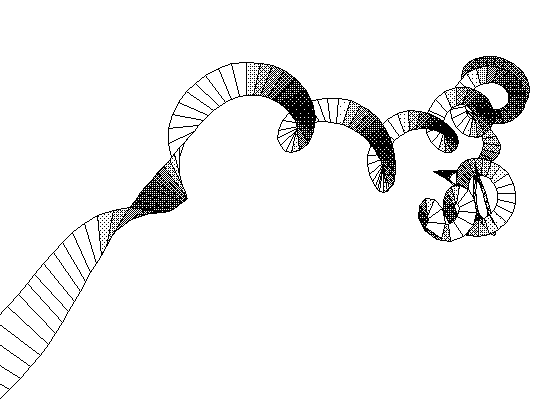#### References:

1. Morris Kline, Mathematical Thought From Ancient To Modern Times, Oxford U. Press, New York, 1972, p. 557.
2. Richard Thompson, "Designing a Baseball Cover," at http://www.mathsoft.com/asolve/baseball/baseball.html, 1996.
3. Yung-Chow Wong, "On An Explicit Characterization of Spherical Curves," Proceedings of the American Mathematical Society 34 (July, 1972), pp. 239-242.
4. Dirk J. Struik, Lectures on Classical Differential Geometry, Addison-Wesley, Reading, Mass, 1961.
5. Rudy Rucker, "Kappa Tau Curves Download Page," at http://www.rudyrucker.com/oldhomepage/kappatau.htm, first posted 1997.
 Home         Biography         Books         Paintings & Links         Classes         Email